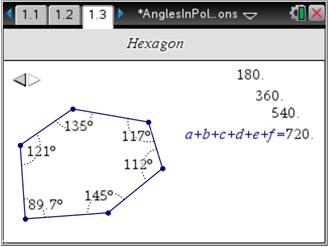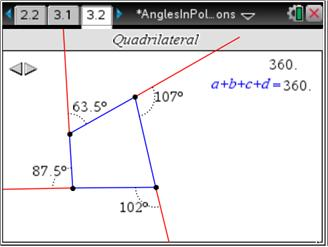# Activities

••• ##### Subject Area

• Math: Geometry: Quadrilaterals and Polygons

• ##### Author9-12

45 Minutes

• ##### Device
•TI-Nspire™ CX/CX II
•TI-Nspire™ CX CAS/CX II CAS
• TI-Nspire™ Navigator™
• TI-Nspire™
• TI-Nspire™ CAS
• ##### Software

TI-Nspire™
TI-Nspire™ CAS

3.2

## Angles in Polygons

#### Activity Overview

In this activity, students measure interior angles in convex polygons and find the sum of the angle measures. They make and test a conjecture about the sum of the angle measures in an n-sided polygon. Finally, they measure exterior angles in convex polygons, find their sum, and write a proof for the sum of the exterior angles.

#### Key Steps

•In this activity, students measure interior angles in convex polygons and use the Calculate tool to find the sum of the angle measures. They keep a record of the sums and make and test a conjecture about the sum of the angle measures in an n-sided polygon.

•Students will also measure exterior angles in convex polygons and find their sum. They will use this knowledge to complete a two-column proof about the sum of the exterior angles.